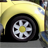# Export list of items within enterprise database

70
1
05-09-2020 03:30 AMNew Contributor II

Hello

I am trying to export a list of items within a enterprise geo-database to excel.  I have not used python before or model builder so any guidance on where to start would be much appreciated.

I would love to be able to get the feature class name, the number of records and the type of the feature class, i.e point, area, polyline etc.

Is the above possible???

Thanks

Andy

1 ReplybyMVP Frequent Contributor

It should work through the python window if you're connected to the sde (I guess).  Hopefully someone with more ken can solve that one if not.

``import arcpyimport osworkspace = r'your sde here'walk = arcpy.da.Walk(workspace, datatype="FeatureClass", type="Polygon")polygon_list = []for dirpath, dirnames, filenames in walk:    for filename in filenames:        polygon_list.append([os.path.join(dirpath, filename), filename])walk = arcpy.da.Walk(workspace, datatype="FeatureClass", type="Polyline")line_list = []for dirpath, dirnames, filenames in walk:    for filename in filenames:        line_list.append([os.path.join(dirpath, filename), filename])walk = arcpy.da.Walk(workspace, datatype="FeatureClass", type="Point")point_list = []for dirpath, dirnames, filenames in walk:    for filename in filenames:        point_list.append([os.path.join(dirpath, filename), filename])print(line_list)for polygon in polygon_list:        row_count = arcpy.GetCount_management(polygon)    print("Polygon -- " + polygon + " -- " + str(row_count))print("\n")for line in line_list:        row_count = arcpy.GetCount_management(line)    print("Line -- " + line + " -- " + str(row_count))print("\n")for point in point_list:        row_count = arcpy.GetCount_management(point)    print("Point -- " + point + " -- " + str(row_count))‍‍‍‍‍‍‍‍‍‍‍‍‍‍‍‍‍‍‍‍‍‍‍‍‍‍‍‍‍‍‍‍‍‍‍‍‍‍‍‍‍‍‍‍‍‍‍‍‍‍‍‍``